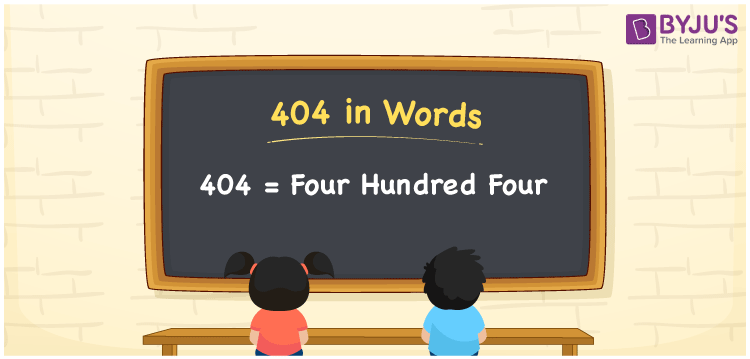# 404 in Words

404 in words is written as Four hundred four. In both the International System of Numerals and the Indian System of Numerals, 404 is written as Four hundred four. The number 404 is a Cardinal Number as it represents some quantity. For example, “404 students have participated in the test”.

 404 in Words Four hundred four Four hundred four in Number 404

## 404 in English Words

We write 404 in English Words using the letters of the English alphabet. Therefore, we read 404 in English as “Four hundred four.”## How to Write 404 in Words?

To write 404 in words, we shall use the place value chart. In the place value chart, write 4 in the hundreds, 0 in the tens, and 4 in the ones, respectively. Now let us make a place value chart to write the number 404 in words.

 Hundreds Tens Ones 4 0 4

Thus, we can write the expanded form as

4 × Hundred + 0 × Ten + 4 × One

= 4 × 100 + 0 × 10 + 4 × 1

= 400 + 0 + 4

= 404

= Four hundred four.

404 is a natural number, the successor of 403 and the predecessor of 405.

404 in words – Four hundred four

• Is 404 an odd number? – No
• Is 404 an even number? – Yes
• Is 404 a perfect square number? – No
• Is 404 a perfect cube number? – No
• Is 404 a prime number? – No
• Is 404 a composite number? – Yes

## Frequently Asked Questions on 404 in Words

Q1

### How to write 404 in words?

404 in words is written as Four hundred four.
Q2

### How to write 404 in the International and Indian System of Numerals?

In both, the system of numerals, 404 in words, is written as Four hundred four.
Q3

### What is the preceding number of 404?

The number that precedes 404 is 403.